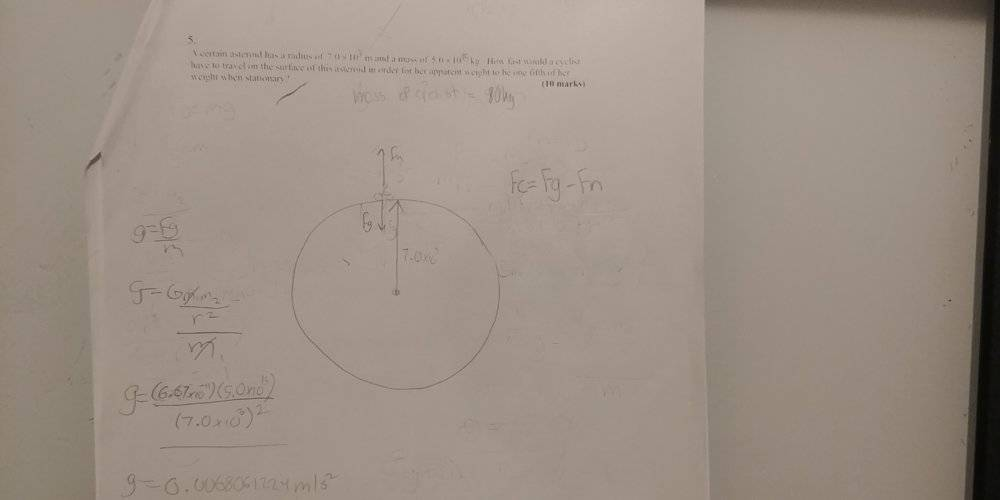# [Grade 12 Physics] Gravitational/circular motion #2

Homework Statement:
A certain asteroid has a radius of 7.0x10^3 m and a mass of 5.0x10^15 kg. How fast would a cyclist have to travel on the surface of this asteroid in order for her apparent weight to be one-fifth of her weight when stationary.
Relevant Equations:
Fcentripetal = ma
a = v^2 / r
Fg = GMm / r^2

Potential Energy = -GMm / r
Kinetic Energy = 0.5(mV^2)
Total Energy (Ek + Ep) = -0.5(GMm) / r

g = Fg / m
Diagram for question 2:Please check if my work is correct.
Main equation: Fc = Fg - Fn
Fn = Fg - Fc
I assume that: Fn = Fg when stationary
Fg = mg
I divide by 5 because the apparent weight must be one fifth of her weight when stationary
Fg = mg / 5
Fn = Fg
Fn = mg / 5
Now to substitute back into the equation Fn = Fg - Fc
Fn / m = g - V^2 / r

Fn(r) / m = g(r) - V^2

mgr / 5m = gr - V^2

-(gr / 5) + gr = V^2

V = 6.17 m/s^2

## Answers and Replies

TSny
Homework Helper
Gold Member
Your work looks correct to me. However, I found the following lines a little confusing:

I assume that: Fn = Fg when stationary
Fg = mg
I divide by 5 because the apparent weight must be one fifth of her weight when stationary
Fg = mg / 5
Fn = Fg
Fn = mg / 5

You got to the correct result: Fn = mg/5. But I didn't quite follow your logic.

The apparent weight is given by the normal force Fn. And you want this to be 1/5 of the actual weight. Hence,
Fn = mg/5. Maybe that's what you were thinking?

I actually didn't know that the apparent weight was Fn but it turned out to be correct. What even is "apparent weight" I thought it was the weight when moving.

FactChecker
Science Advisor
Gold Member
I actually didn't know that the apparent weight was Fn but it turned out to be correct. What even is "apparent weight" I thought it was the weight when moving.
Your phrase "weight when moving" is vague. Many would say that it is still the attraction due to gravity, which is not changed by the motion. The problem says "apparent weight" to imply ##F_n##.

kuruman
Science Advisor
Homework Helper
Gold Member
I actually didn't know that the apparent weight was Fn but it turned out to be correct. What even is "apparent weight" I thought it was the weight when moving.
Weight is the force with the which the Earth, or the asteroid in this case, attracts the object. Apparent weight is the force that a bathroom scale would measure. Contrary to popular belief, a bathroom scale measures the normal force exerted on its surface, not the weight of an object placed on it. When you push on a bathroom scale with your hand, it does not display your weight, does it?

Thus, if you stand on a bathroom scale in a moving elevator, it will display more than your weight if the elevator is accelerating up, less than your weight if accelerating down and your weight if moving at constant speed up or down or is at rest. The same situation applies here except that the normal force is different from the weight not because the mass is accelerating in a straight line but because it is going around in a circular path while maintaining contact with the surface.